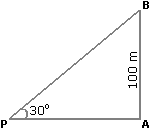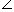# Aptitude - Height and Distance - Discussion

Discussion Forum : Height and Distance - General Questions (Q.No. 5)
5.
From a point P on a level ground, the angle of elevation of the top tower is 30°. If the tower is 100 m high, the distance of point P from the foot of the tower is:
149 m
156 m
173 m
200 m
Explanation:

Let AB be the tower.Then,APB = 30° and AB = 100 m.

 AB = tan 30° = 1 AP 3AP = (AB x 3) m = 1003 m = (100 x 1.73) m = 173 m.

Discussion:
26 comments Page 1 of 3.

Bala murali said:   10 months ago
Here, tan 30 is 1/√3.

Then how will 100/√3 become 1.73?
(2)

Sagar said:   1 year ago
1.73 how?

Vivek Sunny said:   2 years ago
Approach 1*1= 1,
1.5*1.5=2.25,
2*2=4,
so.

√ 3 is between 1.5 and 2 so 1.73 is correct.

Rutuja said:   2 years ago
how Root 3 is calculated as 1.73?

Vikas said:   2 years ago
Why tan only?

Because we find the height of the tower that we get tan30 because;

tan30 = perpendicular/base.

Mounika said:   2 years ago

Nissipravalika said:   3 years ago
Hi,

How to calculate √3 value?

Gitanshu said:   3 years ago
How to divide 34.64/1.732 without calc. Is there any simple trick?

Priya said:   4 years ago
Thank you so much @Sarthak.

You explained it neat and clear.

Vansh giroh said:   4 years ago
Here AP needs to find so using the AB value Tan 30 is taken and root 3 is 1.73. So the answer will be 173m.